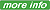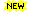Processing ......FreeComputerBooks.com Links to Free Computer, Mathematics, Technical Books all over the World

Number TheoryAlgebra and Linear Algebra BooksCalculus and Mathematical Analysis BooksA Computational Introduction to Number Theory and Algebra ©2005-2009 (Victor Shoup)Algorithms for Modular Elliptic Curves, 2nd Edition (J. E. Cremona)Algorithmic Number Theory: Lattices, Number Fields, Curves and Cryptography ©2008 (Joseph P. Buhler, et al)Number: The Language of Science ©2007 (Tobias Dantzig) 416 pagesEssays on the Theory of Numbers by Richard DedekindAlgebraic Number Theory and Class Field TheoryA Course In Algebraic Number Theory (Robert B. Ash)An Introduction to the Theory of Numbers (Leo Moser)Number Theory Notes for Students at IMO Level ©1995 (Naoki Sato)Number Theory ©2008 (Bruce Ikenaga)Algebraic Number Theory (J.S. Milne)Elementary Number Theory ©2002 (W. Edwin Clark)Elementary Number Theory ©2002 (Michael Filaseta)All CategoriesRecent BooksMiscellaneous BooksComputer LanguagesComputer ScienceData Science/DatabasesElectrical EngineeringJava and Java EE (J2EE)Linux and UnixMathematicsMicrosoft and .NETMobile ComputingNetworking and CommunicationsSoftware EngineeringSpecial TopicsWeb Programming